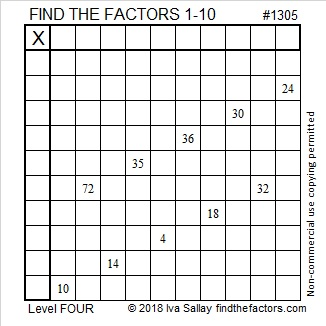# 1305 and Level 4

This level 4 puzzle has clues taken from a standard multiplication table, but the factors of those clues are not in their usual places. Can you figure out where the numbers 1 to 10 belong in this multiplication table?Print the puzzles or type the solution in this excel file: 10-factors-1302-1310

Now I’ll share some information about the puzzle’s number, 1305:

• 1305 is a composite number.
• Prime factorization: 1305 = 3 × 3 × 5 × 29, which can be written 2905 = 3² × 5 × 29
• The exponents in the prime factorization are 2, 1, and 1. Adding one to each and multiplying we get (2 + 1)(1 + 1)(1 + 1) = 3 × 2 × 2 = 12. Therefore 1305 has exactly 12 factors.
• Factors of 1305: 1, 3, 5, 9, 15, 29, 45, 87, 145, 261, 435, 1305
• Factor pairs: 1305 = 1 × 1305, 3 × 435, 5 × 261, 9 × 145, 15 × 87, or 29 × 45
• Taking the factor pair with the largest square number factor, we get √1305 = (√9)(√145) = 3√145 ≈ 36.12478.1305 is the sum of two squares in two ways:
36² + 3² = 1305
27² + 24² = 1305

153-1296-1305 calculated by 27² – 24², 2(27)(24), 27² + 24²
216-1287-1305 calculated by 2(36)(3), 36² – 3², 36² + 3²
783-1044-1305 which is (3-4-5) times 261
900-945-1305 which is (20-21-29) times 45